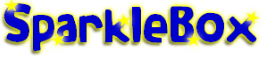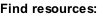﻿ Other Early Years & KS1 Calculating Teaching Resources - SparkleBox

HOME > Maths > Calculations > Other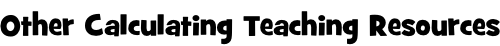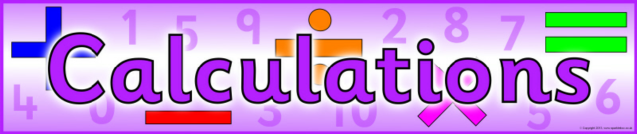Calculations Display Banner (SB100116)

A printable banner for your classroom ‘Calculations’ display board.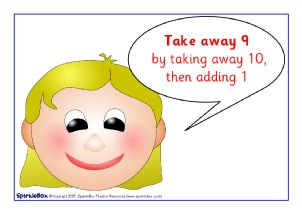Adding and Subtracting 9 and 11 Posters (SB691)

4 very simple posters reminding children of the easy way to add and subtract 9 or 11.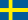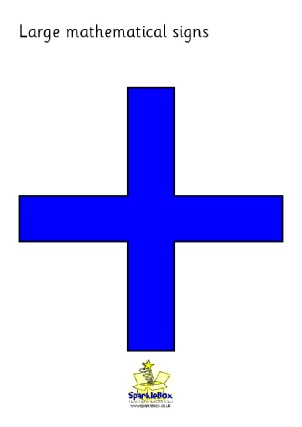Large Mathematical Symbol Signs (SB147)

The main mathematical symbols arranged on A4 in both colour and black.  +, –, =, x and ÷.  Great for use in large-scale number sentences or for hanging in your classroom.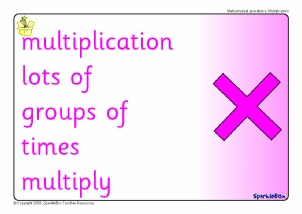Mathematical Vocabulary Posters (SB69)

A colour-coded set of posters featuring the common mathematical operations and words/questions linked to each.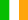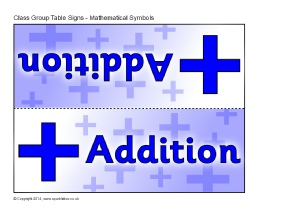Mathematical Symbols Group Table Signs (SB10429)

Print out these foldable tabletop signs for use with your Maths classroom groupings.  Print on card, laminate and fold.  Ideal for Literacy groups.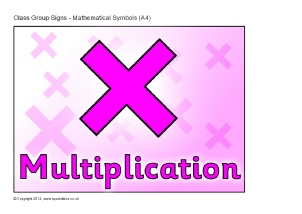Mathematical Symbols Group Signs - A4 (SB10430)

Print out these A4 signs for use on your class Maths group displays.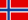Advertisements

Mathematical Operations Banners (SB3145)

5 colour banners featuring the main mathematical operations and associated vocabulary.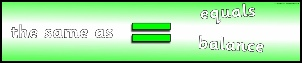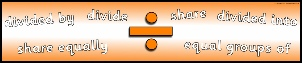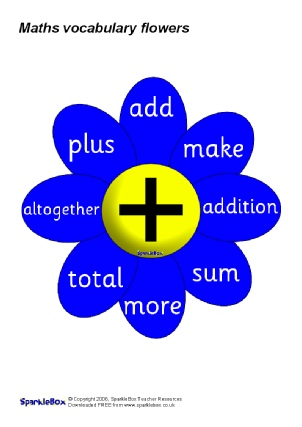Mathematical Vocabulary Flowers (SB403)

A colour-coded set of A4 flowers, each showing a mathematical sign in the centre and the related vocabulary on the petals.

CLASSROOM PHOTO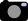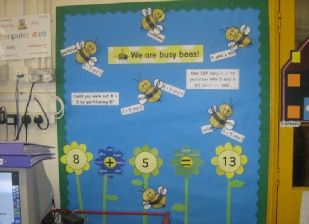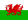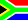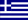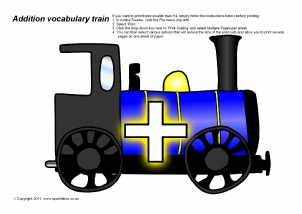Addition Vocabulary Train (SB4079)

A printable engine and carriages with vocabulary for addition.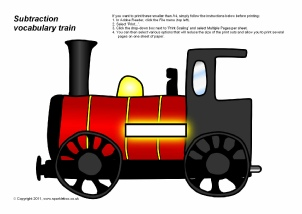Subtraction Vocabulary Train (SB4080)

A printable engine and carriages with vocabulary for subtraction.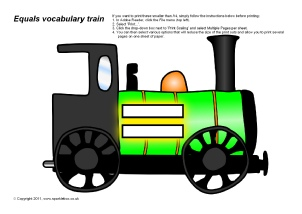Equals Vocabulary Train (SB4081)

A printable engine and carriages with vocabulary for equals.Advertisements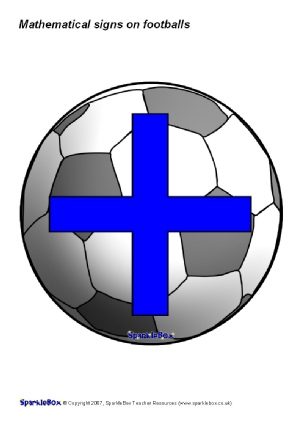Mathematical Symbols on Footballs (SB1018)

The main mathematical symbols arranged on A4-sized footballs.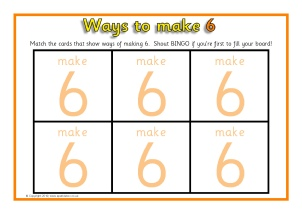Ways to Make a Total Bingo (SB3346)

Bingo boards and cards with various ways of making totals from 1 to 6.  Children match the calculation cards to their board.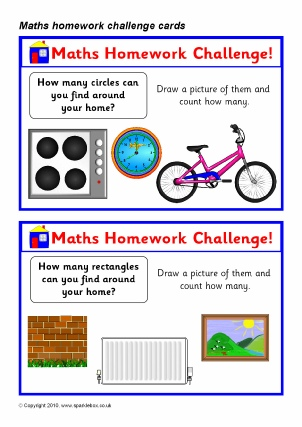Maths Homework Challenge Cards (SB3268)

A set of 30 homework activity cards for children to take home.  Includes tasks such as finding who has the largest hands in the family, adding numbers on a car number plate, counting activities, 2D shape hunts and much more.

Number Facts Display Banner (SB5921)

A colour banner for your ‘Number Facts’ classroom display.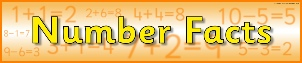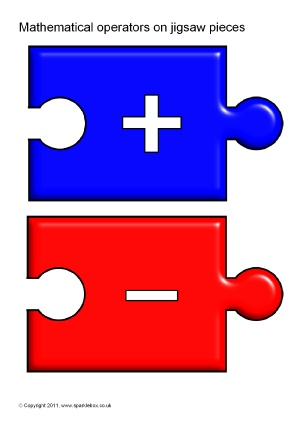Mathematical Operators on Jigsaw Pieces (SB1101)

Coloured jigsaw pieces featuring the basic mathematical operators.  Great for use along with our Numbers 0-50 on jigsaw pieces to assemble number sentences.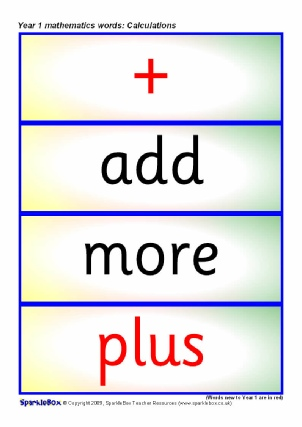Year 1 Maths Vocabulary Cards: Calculations (SB2236)

A set of printable cards featuring the mathematical vocabulary for ‘Calculations’ to be used in Year One.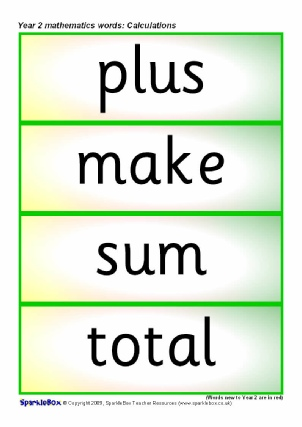Year 2 Maths Vocabulary Cards: Calculations (SB2256)

A set of printable cards featuring the mathematical vocabulary for ‘Calculations’ to be used in Year Two.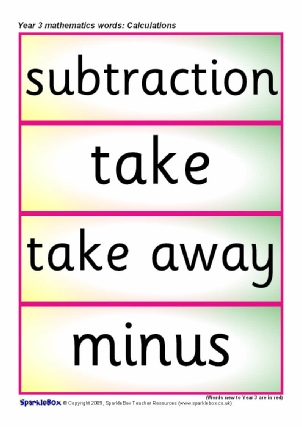Year 3 Maths Vocab Cards: Calculations (SB6882)

A set of printable cards featuring the mathematical vocabulary for ‘Calculations’ to be used in Year 3.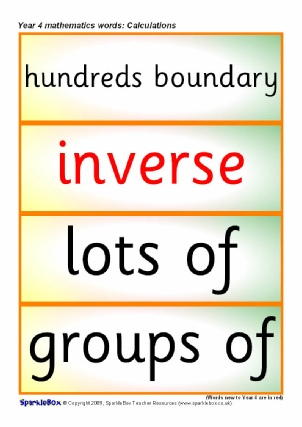Year 4 Maths Vocab Cards: Calculations (SB6898)

A set of printable cards featuring the mathematical vocabulary for ‘Calculations’ to be used in Year 4.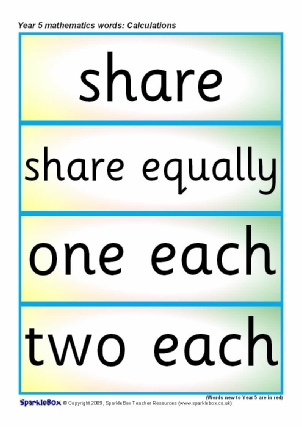Year 5 Maths Vocab Cards: Calculations (SB6905)

A set of printable cards featuring the mathematical vocabulary for ‘Calculations’ to be used in Year 5.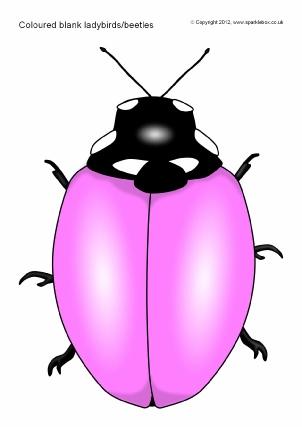Blank Coloured Ladybirds/Beetles (SB7971)

A set of A4-sized blank (no spots) ladybirds/beetles in a variety of colours.  Great for all sorts of uses: maths counter games, symmetry activities, pupil sticker (spots) reward cards, colour recognition and more!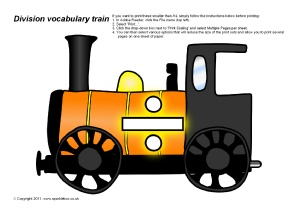Division Vocabulary Train (SB4083)

A printable engine and carriages with vocabulary for division.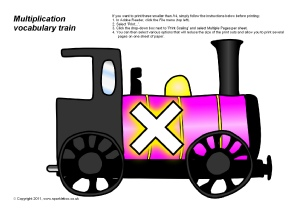Multiplication Vocabulary Train (SB4082)

A printable engine and carriages with vocabulary for multiplication.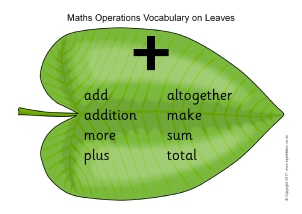Mathematical Vocabulary on Leaves (SB11961)

Printable A4-sized leaves featuring vocabulary related to each of the main mathematical operations.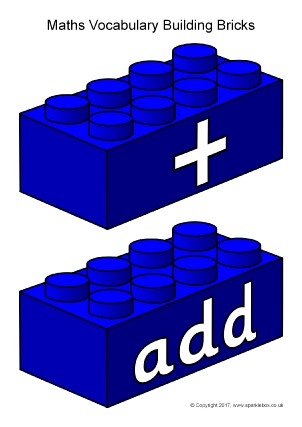Mathematical Vocabulary on Building Bricks (SB12064)

A colour-coded set of connecting brick images featuring words associated with the four main mathematical operations.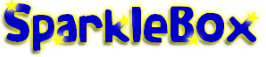Follow us:

© Copyright SparkleBox Teacher Resources (UK) Ltd.  About Us | Terms and Conditions of Use | Copyright | Privacy Policy | Cookie Policy | FAQ

New Literacy Maths Signs and Labels Class Management Special Needs Other Topics